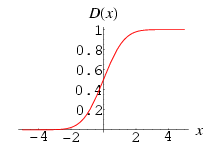•http://facebook.com/
•https://www.google.com/accounts/o8/id
•https://me.yahoo.comCOST (GBP)0.100.800

# CDF

viewed 1941 times and licensed 63 times
Evaluates the standard normal cumulative density function.
Contents## DependentsC++

## CDF

 doubleCDF( double x )[inline]
This function evaluates the standard normal cumulative density function, which is the area under the Gaussian probability density function, integrated over the range : where and erf is the error function computing using errorFn.

This function has the following form:### Example 1

#include <iostream>
#include <codecogs/stats/dists/continuous/stdnormal/cdf.h>
using namespace std;
int main()
{
cout << "standard normal CDF (0.3) = ";
cout << Stats::Dists::Continuous::StdNormal::CDF(0.3) << endl;
return 0;
}
Output:
standard normal CDF (0.3) = 0.617911

### References

http://mathworld.wolfram.com/StandardNormalDistribution.html

### Parameters

 x The upper limit on the integral

### Authors

Lucian Bentea (September 2005)
##### Source Code

Source code is available when you agree to a GP Licence or buy a Commercial Licence.

Not a member, then Register with CodeCogs. Already a Member, then Login.